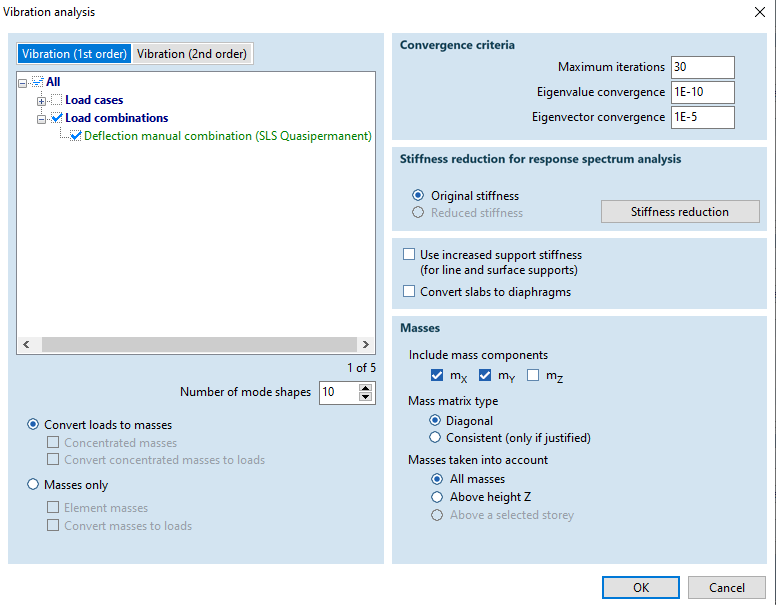# Basics of vibration and seismic analysis

The AXISVM program can be used for analyzing vibrations and for doing seismic calculatins as well. The following lines will illuminate the basic questions of these topics.

# Calculation time and modelling simplifications#

The calculation of natural vibrations uses the (spatial) calculation model. There are no simplifications of this model that are applied automatically (e.g. conversion of surfaces into beams), as the user would not be aware of these simplifications and it would be very difficult to check if they are valid. Optionally it is possible to “convert slabs to diaphragms”, which is not recommended for reasons mentioned above and others.

Diaphragms are completely rigid in their (horizontal) “plane of operation”. Their use to represent floor slabs can accelerate the vibration analysis (mostly for skeletal structures), but it is to be viewed as a simplification of the model. The calculated vibrations for the diaphragm model should be treated as approximation. For seismic calculations with the response spectrum method, this approximations is sufficiently accurate in most cases.

# Optimizing calculation time#

The vibration analysis requires typically a greater calculation time than the linear statical analysis. Depending on the type and size of the model, the calculation time can be reduced more or less drastically with appropriate procedures.

• Modelling
• As simple as possible, as exact as necessary
• Mesh size
• The size of the equation system is strongly depends on the mesh size
• The chosen mesh size influences the number of calculated nodes and therefore the results’ detail level, but not the numerical accuracy of the calculation
• Number of calculated mode shapes, mass components
• Each mode shape is calculated by itself. The calculation time depends more or less linearly on the number of calculated mode shapes
• The respected mass components (direction of inertial forces) influences the number of mode shapes necessary (modal mass ratio). In many cases, the inclusion of the vertical vibrations (mass component mz) is not needed.

# Analysis parameters#

The vibration analysis requires some parameters. The following lines contain the detailing of this.• Vibration according to theory 1st or 2nd order
• 1st order analysis is standard for seismic analysis, but it neglects the effect of normal forces regarding the stiffness
• 2nd order analysis has an influence of normal forces on the natural frequencies
• Compression → lower frequency, the stiffness decreases
• Tension → higher frequency, the stiffness increases
• A linear static analysis is required to determinate the normal forces
• Number of mode shapes – the number of calculated mode shapes is given by the user, default value is 9
• Respected Masses
• Convert loads to masses – the load parallel with the gravitation of the selected load case and/or load combination are converted automatically to masses (standard case)
• Masses only – loads are neglected for the vibration analysis
• Mass components – the mass components represent the direction of vibrations to be considered. For seismic analysis, the mass component mz (vertical share of vibration) can be neglected in many cases
• Mass matrix type
• Diagonal – standard case, without centrifugal inertias
• Consistent – total mass matrix, considers centrifugal inertias (generally not recommended)
• Masses taken into account – it is adjustable which levels’ masses the user wants to take into account
• Diaphragms – automatic conversion of adequate slabs (without surface support, etc.) to diaphragms – mostly useful in case of skeletal structures
• Convergence criteria – settings for the accuracy of the calculation. Changes to these values require detailed knowledge of the mathematical calculation process

Source: http://www.ingware.com